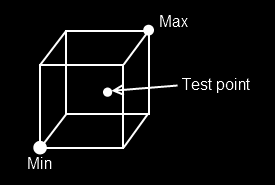# Point in AABB

Figuring out if a point is inside of an AABB is pretty simple, especially if you think of an AABB as being represented by a min and a max point. Take a look at the image below and try to figure out the algorithm before i describe it in the next paragraph### The algorithm

Looking at the above image, we can figure out that if a point is inside of a AABB:

• The relationship of point to min is such:
• Point.X, Point.Y and Point.Z are all greater than Min.X, Min.Y and Min.Z.
• The relationship of point to max is such:
• Point.X, Point.Y and Point.Z are all less than Min.X, Min.Y and Min.Z.

That is to say, if Point > min && Point < max then the point is inside the AABB.

Add the following function to the Collisions class:

public static bool PointInAABB(AABB aabb, Point point)


And provide an implementation for it!

### Unit Test

The sample / Unit test code for this section will be included with the next (Closest point on AABB) section.